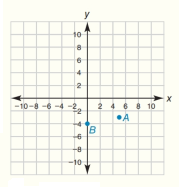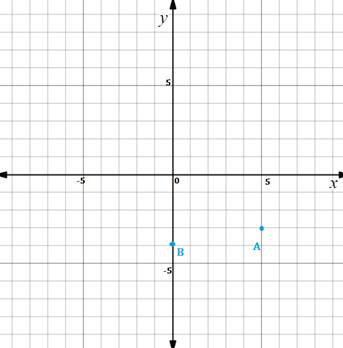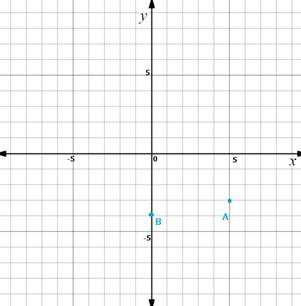Chapter 10.CT, Problem 1CTElementary Geometry For College St...

7th Edition
Alexander + 2 others
ISBN: 9781337614085

Solutions

Chapter
SectionElementary Geometry For College St...

7th Edition
Alexander + 2 others
ISBN: 9781337614085
Textbook Problem

In the coordinate system provided, give the coordinates ofa) point A in the form x ,   y . a) point B in the form x ,   y .To determine

(a)

To find:

The coordinates of the point A in the form x, y.

Solution:

The coordinates of the point A=(5, -3).

Explanation

Given figure is,Point A is located at a distance from origin in the x-axis =5

Point A is located at a distance from origin in the y-axis =-3

Hence, the coordinates of the point A=(5, -3).

Final statement:

The coordinates of the point A=(5, -3).

To determine

(b)

To find:

The coordinates of the point B in the form x, y.

Solution:

The coordinates of the point B=(0, -4).

Explanation

Given figure is,Point A is located at a distance from origin in the x-axis =0

Point A is located at a distance from origin in the y-axis =-4

Hence, the coordinates of the point B=(0, -4).

Final statement:

The coordinates of the point B=(0, -4).

The Solution to Your Study Problems

Bartleby provides explanations to thousands of textbook problems written by our experts, many with advanced degrees!

Get Started

Change 40 mi/h to ft/s.

Elementary Technical Mathematics

limx(12)x2= a) 0 b) 1 c) d) does not exist

Study Guide for Stewart's Single Variable Calculus: Early Transcendentals, 8th

True or False: is a convergent series.

Study Guide for Stewart's Multivariable Calculus, 8th

Find all the answers to your study problems with bartleby.
Textbook solutions plus Q&A. Get As ASAP arrow_forward